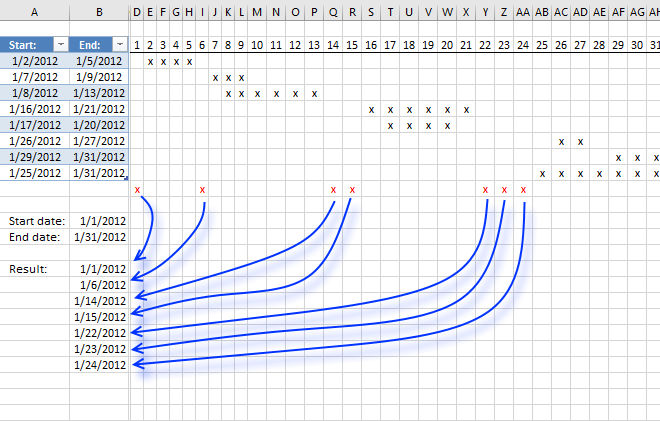Author: Oscar Cronquist Article last updated on December 22, 2018The Excel defined table contains start and end dates for each date range in cell range A3:B10. Cell B13 is the start date and B14 is the end date which are the outer boundaries, obviously, we can't list all dates that ever existed.

The array formula in cell B16 filters all dates between the start and end date and outside the specified date ranges in the Excel defined table.

I made a simple calendar (D3:AH10) next to the Excel defined table (A3:B10) that shows the date ranges and dates not in date ranges (red x). Row 2 contains the days in January 1 to 31, each x below row 2 represents a day in each date range. This makes it much easier to demonstrate and explain what the formula does and also verify the formula result.

Array formula in cell B16:

=IFERROR(SMALL(IF(COUNTIFS(Table1[Start:], "<="&\$B\$13+ROW(\$A\$1:INDEX(\$A:\$A, \$B\$14-\$B\$13))-1, Table1[End:], ">="&\$B\$13+ROW(\$A\$1:INDEX(\$A:\$A, \$B\$14-\$B\$13))-1), "", \$B\$13+ROW(\$A\$1:INDEX(\$A:\$A, \$B\$14-\$B\$13))-1), ROW(A1)), "")

How to create an array formula

1. Copy above array formula
2. Click in formula bar
3. Paste array formula (Ctrl + v)
4. Press and hold Ctrl + Shift
5. Press Enter

How to copy array formula

1. Select cell B16
2. Copy cell (Ctrl + c)
3. Select cell range B17:B25
4. Paste (Ctrl + v)

### Explaining formula in cell B16

#### Step 1 - Dynamic cell reference

The INDEX function creates a cell reference based on cell B14 - B13. This cell referenc will in a later step be used to create an array containing a sequence of numbers ranging from 0 to 29. If you change the dates in cell B13 or B14 a new sequence of values is instantly created.

\$A\$1:INDEX(\$A:\$A,\$B\$14-\$B\$13)

becomes

\$A\$1:INDEX(\$A:\$A,40939-40909)

becomes

\$A\$1:INDEX(\$A:\$A,30)

and returns

\$A\$1:\$A\$30

#### Step 2 - Create a sequence and add a less than sign to each value in the array

"<="&\$B\$13+ROW(\$A\$1:INDEX(\$A:\$A,\$B\$14-\$B\$13))-1

becomes

"<="&\$B\$13+ROW(\$A\$1:\$A\$30)-1

becomes

"<="&\$B\$13+{0; 1; 2; 3; 4; 5; 6; 7; 8; 9; 10; 11; 12; 13; 14; 15; 16; 17; 18; 19; 20; 21; 22; 23; 24; 25; 26; 27; 28; 29}

becomes

"<="&40909+{0; 1; 2; 3; 4; 5; 6; 7; 8; 9; 10; 11; 12; 13; 14; 15; 16; 17; 18; 19; 20; 21; 22; 23; 24; 25; 26; 27; 28; 29}

becomes

"<="&{40909; 40910; 40911; 40912; 40913; 40914; 40915; 40916; 40917; 40918; 40919; 40920; 40921; 40922; 40923; 40924; 40925; 40926; 40927; 40928; 40929; 40930; 40931; 40932; 40933; 40934; 40935; 40936; 40937; 40938}

and returns

{"<=40909"; "<=40910"; "<=40911"; "<=40912"; "<=40913"; "<=40914"; "<=40915"; "<=40916"; "<=40917"; "<=40918"; "<=40919"; "<=40920"; "<=40921"; "<=40922"; "<=40923"; "<=40924"; "<=40925"; "<=40926"; "<=40927"; "<=40928"; "<=40929"; "<=40930"; "<=40931"; "<=40932"; "<=40933"; "<=40934"; "<=40935"; "<=40936"; "<=40937"; "<=40938"}

#### Step 3 - Check if dynamic dates are inside the date ranges

The COUNTIFS function calculates the number of cells across multiple ranges that equals all given conditions. The date ranges has a start date and an end date, that means we need two conditions to check if dates are inside the date ranges. The only difference between these two conditions are the less than and greater than signs concatenated to each date.

COUNTIFS(Table1[Start:], "<="&\$B\$13+ROW(\$A\$1:INDEX(\$A:\$A, \$B\$14-\$B\$13))-1, Table1[End:], ">="&\$B\$13+ROW(\$A\$1:INDEX(\$A:\$A, \$B\$14-\$B\$13))-1)

returns

{0; 1; 1; 1; 1; 0; 1; 2; 2; 1; 1; 1; 1; 0; 0; 1; 2; 2; 2; 2; 1; 0; 0; 0; 1; 2; 2; 1; 2; 2}.

#### Step 4 - Replace 0 (zero) in array with corresponding date

The IF function has three arguments, the first one must be a logical expression. If the expression evaluates to TRUE then one thing happens (argument 2) and if FALSE another thing happens (argument 3).

IF(COUNTIFS(Table1[Start:], "<="&\$B\$13+ROW(\$A\$1:INDEX(\$A:\$A, \$B\$14-\$B\$13))-1, Table1[End:], ">="&\$B\$13+ROW(\$A\$1:INDEX(\$A:\$A, \$B\$14-\$B\$13))-1), "", \$B\$13+ROW(\$A\$1:INDEX(\$A:\$A, \$B\$14-\$B\$13))-1)

becomes

IF({0; 1; 1; 1; 1; 0; 1; 2; 2; 1; 1; 1; 1; 0; 0; 1; 2; 2; 2; 2; 1; 0; 0; 0; 1; 2; 2; 1; 2; 2}, "", {40909; 40910; 40911; 40912; 40913; 40914; 40915; 40916; 40917; 40918; 40919; 40920; 40921; 40922; 40923; 40924; 40925; 40926; 40927; 40928; 40929; 40930; 40931; 40932; 40933; 40934; 40935; 40936; 40937; 40938})

becomes

IF({0; 1; 1; 1; 1; 0; 1; 2; 2; 1; 1; 1; 1; 0; 0; 1; 2; 2; 2; 2; 1; 0; 0; 0; 1; 2; 2; 1; 2; 2}, "", \$B\$13+ROW(\$A\$1:INDEX(\$A:\$A, \$B\$14-\$B\$13))-1)

and returns

{40909; ""; ""; ""; ""; 40914; ""; ""; ""; ""; ""; ""; ""; 40922; 40923; ""; ""; ""; ""; ""; ""; 40930; 40931; 40932; ""; ""; ""; ""; ""; ""}

#### Step 5 - Extract k-th smallest date

To be able to return a new value in a cell each I use the SMALL function to filter date numbers from smallest to larges

SMALL(IF(COUNTIFS(Table1[Start:], "<="&\$B\$13+ROW(\$A\$1:INDEX(\$A:\$A, \$B\$14-\$B\$13))-1, Table1[End:], ">="&\$B\$13+ROW(\$A\$1:INDEX(\$A:\$A, \$B\$14-\$B\$13))-1), "", \$B\$13+ROW(\$A\$1:INDEX(\$A:\$A, \$B\$14-\$B\$13))-1), ROW(A1))

becomes

SMALL({40909; ""; ""; ""; ""; 40914; ""; ""; ""; ""; ""; ""; ""; 40922; 40923; ""; ""; ""; ""; ""; ""; 40930; 40931; 40932; ""; ""; ""; ""; ""; ""}, ROW(A1))

becomes

SMALL({40909; ""; ""; ""; ""; 40914; ""; ""; ""; ""; ""; ""; ""; 40922; 40923; ""; ""; ""; ""; ""; ""; 40930; 40931; 40932; ""; ""; ""; ""; ""; ""}, 1)

and returns 40909 (1/1/2012) in cell B16.

#### Step 6 - Replacce errors with blanks

When there are no more values to extract the formula returns errors, the IFERROR function removes the errors and returns blank cells.

IFERROR(SMALL(IF(COUNTIFS(Table1[Start:], "<="&\$B\$13+ROW(\$A\$1:INDEX(\$A:\$A, \$B\$14-\$B\$13))-1, Table1[End:], ">="&\$B\$13+ROW(\$A\$1:INDEX(\$A:\$A, \$B\$14-\$B\$13))-1), "", \$B\$13+ROW(\$A\$1:INDEX(\$A:\$A, \$B\$14-\$B\$13))-1), ROW(A1)), "")